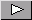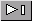Math Central - mathcentral.uregina.caResource RoomResource RoomStrandSecondary - Algebrastart over

We found 29 items matching your search.Page1/2In this note Gregory describes a problem involving Dasher and Dancer moving around a Northern Light Circle. AUTHOR(S): Gregory V. AkulovA diamond slope, or the slope of the angle bisector, is considered in this note as a generalization of two well-known slope relationships. This general approach is compared then with well-known approaches using various examples. AUTHOR(S): Gregory V. AkulovIn this note the authors give an expression for locating the midpoint of a circular arc and a calculator for determining the midpoint. AUTHOR(S): Gregory V. Akulov and Oleksandr (Alex) G. AkulovIn this note the authors give an proof of the expression for locating the midpoint of a circular arc that was given in his note with Gregory V. Akulov. AUTHOR(S): Oleksandr (Alex) G. AkulovGregory and Oleksandr have built on the arc midpoint resource and the proof of the arc midpoint formula by constructing an algorithm for finding the coordinates of the midpoint. It is hoped that teachers of high school Mathematics and Computer Science will use these resources to enrich the teaching and learning in both subject areas. AUTHOR(S): Oleksandr G. Akulov and Gregory V. AkulovGregory and Oleksandr use their Arc Midpoint Computation approach to solve a problem concerning gravitational potential energy and then challenge the reader to solve the same problem using an alternative approach. AUTHOR(S): Gregory V. Akulov and Oleksandr G. AkulovGregory and Oleksandr extend their arc midpoint computation to determine the midpoint of a section of a sine curve. AUTHOR(S): Gregory V. Akulov and Oleksandr G. AkulovGregory finds another application of his arc midpoint computation, this time to the kinetic energy of an object moving along a semicircle. AUTHOR(S): Gregory V. AkulovOleksandr and Gregory extend interpretations of non-piecewise identities for sin^(-1)x+ sin^(-1)y and cos^(-1)x+ cos^(-1)y using several Euclidean geometry statements and illustrations. AUTHOR(S): Oleksandr G. Akulov and Gregory V. AkulovThis note is a response to a teacher's request for an explaination of i squared and the square root of i that would be appropriate for secondary level students. AUTHOR(S): Harley WestonIn this note Gregory uses a trig identity to develop an expression for the slopes of the angle bisectors of two lines in terms of the slopes of the lines that form the angle. AUTHOR(S): Gregory V. AkulovA trigonometric identity is used to develop a formula for the slope of a rhombus diagonal. This expression is then used to find the velocity of a whale. AUTHOR(S): Gregory V. Akulov and Oleksii V. AkulovA unit on direct and partial variation that can be taught after the introduction of linear functions. AUTHOR(S): Ray MahThis article is part of the Mathematics Notes series at Washington State University. In the article, Judi and Harley start by determining the functions that map the plane back onto itself, while at the same time, mapping a specified line back onto itself and preserving the size and shape of any objects represented in the plane. These are the functions that preserve frieze patterns. The authors then look at the algebraic structure of this collection of functions under the operation of composition, show that there are only seven frieze groups, and illustrate how they are generated. Each frieze group is represented algebraically and geometrically. The article concludes with a tour of the Washington State University campus, looking at the ways in which frieze groups are exhibited and used in our immediate surroundings. AUTHOR(S): Judith J. McDonald and J. Harley WestonGregory and Oleksandr find more compact and efficient ways to express some identities involving arcsine and arccosine that appear in the Handbook of Mathematics. The expression Gregory and Oleksandr found was used to derive their arc midpoint computation. AUTHOR(S): Gregory V. Akulov and Oleksandr G. AkulovPage1/2Math Central is supported by the University of Regina and The Pacific Institute for the Mathematical Sciences.about math central :: site map :: links :: notre site français